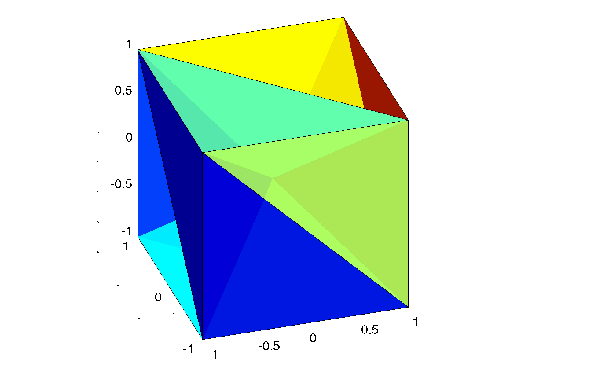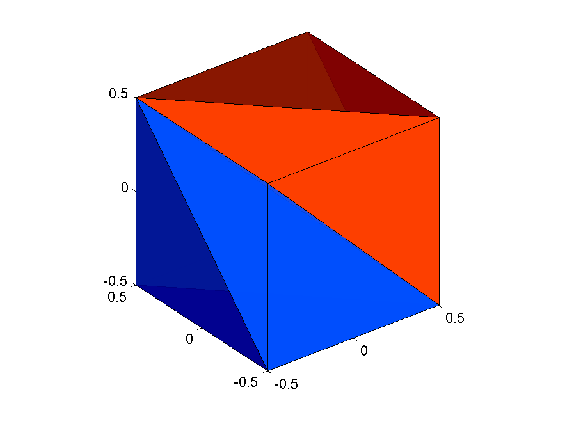MATLAB Function Referencedelaunayn

N-dimensional Delaunay tessellation

Syntax

• ```T = delaunayn(X)
T = delaunayn(X, options)
```

Description

```T = delaunayn(X) ``` computes a set of simplices such that no data points of `X` are contained in any circumspheres of the simplices. The set of simplices forms the Delaunay tessellation. `X` is an `m`-by-`n` array representing m points in n-dimensional space. `T` is a `numt`-by-(`n+1`) array where each row contains the indices into `X` of the vertices of the corresponding simplex.

`delaunayn` uses Qhull.

`T = delaunayn(X, options)` specifies a cell array of strings `options` to be used as options in Qhull. The default options are:

• `{'Qt','Qbb','Qc'}` for 2- and 3-dimensional input
• `{'Qt','Qbb','Qc','Qx'}` for 4 and higher-dimensional input

If `options` is `[]`, the default options used. If `options` is `{''}`, no options are used, not even the default. For more information on Qhull and its options, see http://www.qhull.org.

Visualization

Plotting the output of `delaunayn` depends of the value of `n`:

• For `n = 2`, use `triplot`, `trisurf`, or `trimesh` as you would for `delaunay`.
• For `n = 3`, use `tetramesh` as you would for `delaunay3`.
• You cannot plot `delaunayn` output for `n > 3`.

Examples

Example 1. This example generates an n-dimensional Delaunay tessellation, where `n = 3`.

• ```d = [-1 1];
[x,y,z] = meshgrid(d,d,d);  % A cube
x = [x(:);0];
y = [y(:);0];
z = [z(:);0];
% [x,y,z] are corners of a cube plus the center.
X = [x(:) y(:) z(:)];
Tes = delaunayn(X)

Tes =
```   9  1  5  6
``````   3  9  1  5
``````   2  9  1  6
``````   2  3  9  4
``````   2  3  9  1
``````   7  9  5  6
``````   7  3  9  5
``````   8  7  9  6
``````   8  2  9  6
``````   8  2  9  4
``````   8  3  9  4
``````   8  7  3  9
``````

You can use `tetramesh` to visualize the tetrahedrons that form the corresponding simplex. `camorbit` rotates the camera position to provide a meaningful view of the figure.

• ```tetramesh(Tes,X);camorbit(20,0)```

Example 2. The following example illustrates the `options` input for `delaunayn`.

• ```X = [-0.5 -0.5  -0.5;...
-0.5 -0.5   0.5;...
-0.5  0.5  -0.5;...
-0.5  0.5   0.5;...
0.5 -0.5  -0.5;...
0.5 -0.5   0.5;...
0.5  0.5  -0.5;...
0.5  0.5   0.5];
```

The command

• ```T = delaunayn(X);
```

returns the following error message.

??? qhull input error: can not scale last coordinate. Input is cocircular or cospherical. Use option 'Qz' to add a point at infinity.

This suggests that you add `'Qz'` to the default options.

• ```T = delaunayn(X,{'Qt','Qbb','Qc','Qz'});
```

To visualize this answer you can use the `tetramesh` function:

• ```tetramesh(T,X)
```Algorithm

`delaunayn` is based on Qhull . For information about Qhull, see http://www.qhull.org/. For copyright information, see http://www.qhull.org/COPYING.txt.

See Also

`convhulln`, `delaunayn`, `delaunay3`, `tetramesh`, `voronoin`

Reference

  Barber, C. B., D.P. Dobkin, and H.T. Huhdanpaa, "The Quickhull Algorithm for Convex Hulls," ACM Transactions on Mathematical Software, Vol. 22, No. 4, Dec. 1996, p. 469-483. Available in HTML format at http://www.acm.org/ pubs/citations/journals/toms/1996-22-4/p469-barber/ and in PostScript format at ftp://geom.umn.edu/pub/software/qhull-96.ps.

  National Science and Technology Research Center for Computation and Visualization of Geometric Structures (The Geometry Center), University of Minnesota. 1993.

© 1994-2005 The MathWorks, Inc.